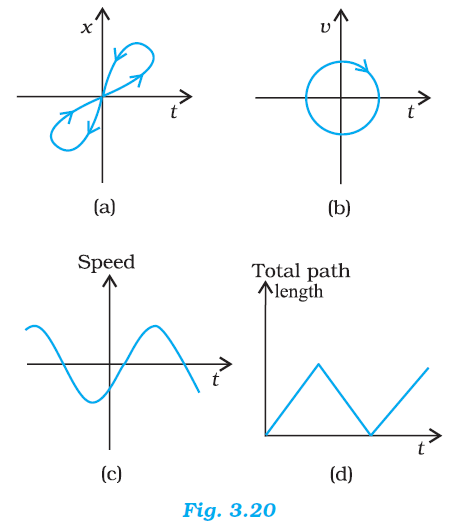## Pages

### Motion in a straight line NCERT Solutions Class 11 Physics - Solved Exercise Question 3.16

Question 3.16

Look at the graphs (a) to (d) (Fig. 3.20) carefully and state, with reasons, which of these cannot possibly represent one-dimensional motion of a particle.Solution:

(a) The given x-t graph, shown in (a), does not represent one-dimensional motion of the particle. This is because a particle cannot have two positions at the same instant of time.

(b) The given v-t graph, shown in (b), does not represent one-dimensional motion of the particle. This is because a particle can never have two values of velocity at the same instant of time.

(c) The given v-t graph, shown in (c), does not represent one-dimensional motion of the particle. This is because speed being a scalar quantity cannot be negative.

(d) The given v-t graph, shown in (d), does not represent one-dimensional motion of the particle. This is because the total path length travelled by the particle cannot decrease with time.